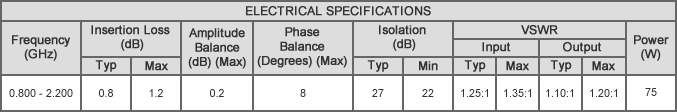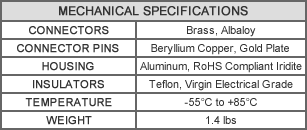# M8N-1.500W, N-Female, 0.8-2.2 GHz

Original price - Original price
Original price
-
Current price
SKU M8N-1.500W

Description:
MECA's 8-way, M8N-1.500W, M-Series N-Female power divider/combiner with an average power rating of 75 watts and frequency of 0.8-2.2 GHz.Notes:
1. All units rated for 75 watts maximum input power as a divider or balanced combiner with load VSWR of 1.20:1 or better.To calculate the combining rated power the following formula is used: (rated input power of divider * 5%) / "N" # of input channels = max input at each port for combining.
2. All output/power combiner ports are in-phase (0° difference).
3. 50 ohm nominal impedance standard.
4. Operating temperature from -55°C to +85°C.
5. See application note. MAP-801